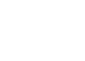﻿ Factorial Notation
FACTORIAL NOTATION
by Dr. Carol JVF Burns (website creator)
Follow along with the highlighted text while you listen!
• PRACTICE (online exercises and printable worksheets)

Recall from the previous section ( Choosing Things: Does Order Matter?)
that $\,{}_nC_k\,$ denotes the number of ways to choose $\,k\,$ items from $\,n\,$ (without replacement) when the order doesn't matter;
it is the number of combinations that are possible when choosing $\,k\,$ items from $\,n\,$ items.

Also, $\,{}_nP_k\,$ denotes the number of ways to choose $\,k\,$ items from $\,n\,$ (without replacement) when the order does matter;
it is the number of permutations that are possible when arranging $\,k\,$ items that are chosen from $\,n\,$ items.

You will see in the next section that the formulas for $\,{}_nC_k\,$ and $\,{}_nP_k\,$ involve patterns like this: $$\cssId{s7}{10 \times 9 \times 8 \times 7 \times 6 \times 5 \times 4 \times 3 \times 2 \times 1}$$

In this pattern, you start with a whole number, multiply by one less than this number, and continue until you get down to $\,1\,$.
It is tedious and inefficient to write down this pattern, particularly when the starting number is large:
$$\cssId{s12}{30 \cdot 29 \cdot 28 \cdot 27 \cdot 26 \cdot 25 \cdot 24 \cdot 23 \cdot 22 \cdot 21 \cdot 20 \cdot 19 \cdot 18 \cdot 17 \cdot 16 \cdot 15 \cdot 14 \cdot 13 \cdot 12 \cdot 11 \cdot 10 \cdot 9 \cdot 8 \cdot 7 \cdot 6 \cdot 5 \cdot 4 \cdot 3 \cdot 2 \cdot 1}$$ Fortunately, there is a compact notation for this pattern, called factorial notation.
We can write: $$\cssId{s15}{10!} \cssId{s16}{= 10 \times 9 \times 8 \times 7 \times 6 \times 5 \times 4 \times 3 \times 2 \times 1}$$ The expression $\,10!\,$ is read aloud as ten factorial.

Remember that multiplication can be denoted in several ways.
In the examples below, the preferred (simplest) way is shown first: $$\begin{gather} \cssId{s20}{3\cdot 2} \cssId{s21}{= 3\times 2} \cssId{s22}{= (3)(2)} \cr \cssId{s23}{ab} \cssId{s24}{= a\cdot b} \cssId{s25}{=a \times b} \cssId{s26}{=(a)(b)} \end{gather}$$

In the definition below, the symbol $\,:=\,$ (an equal sign, with colon in front of it) emphasizes equal, by definition.

DEFINITION factorial notation
Let $\,n\,$ be a whole number; i.e., a member of the set $\,\{0,1,2,3,\ldots\}\,$.

For $\,n\gt 1\,$: $$\cssId{s32}{n! \ :=\ n(n-1)(n-2) \times \cdots \times 1}$$ Also: $$\cssId{s34}{ \begin{gather} 1!\ :=\ 1 \cr 0!\ :=\ 1 \end{gather}}$$ The symbol ‘$\,n!\,$’ is read aloud as ‘$\,n\,$ factorial’.

Another pattern that frequently arises in counting arguments is something like this: $$\cssId{s37}{30 \times 29 \times 28 \times 27 \times 26 \times 25 \times 24}$$ This pattern starts out the same as the pattern for $\,30!\,$ ($\,30\,$ factorial), but you don't continue all the way down to the number $\,1\,$.
Clearly, this pattern can also be tedious and inefficient.

Is there a way to use factorial notation to get a simpler name for this kind of pattern? Yes!
The key is to multiply by $\,1\,$ in an appropriate form, as shown below:

 $30\cdot 29\cdot 28\cdot 27\cdot 26 \cdot 25\cdot 24$ $\displaystyle = 30\cdot 29 \cdot 28\cdot 27\cdot 26\cdot 25\cdot 24 \cdot \frac{23!}{23!}$ (multiply by one in an appropriate form)$= \displaystyle \frac{30\cdot 29\cdot 28\cdot 27 \cdot 26\cdot 25\cdot 24\cdot 23!}{23!}$ (move numbers into the numerator) $\displaystyle = \frac{30!}{23!}$ (Now, we have $\,30\,$ down to $\,1\,$ in the numerator)

This web exercise will give you practice with factorial notation.

Master the ideas from this section